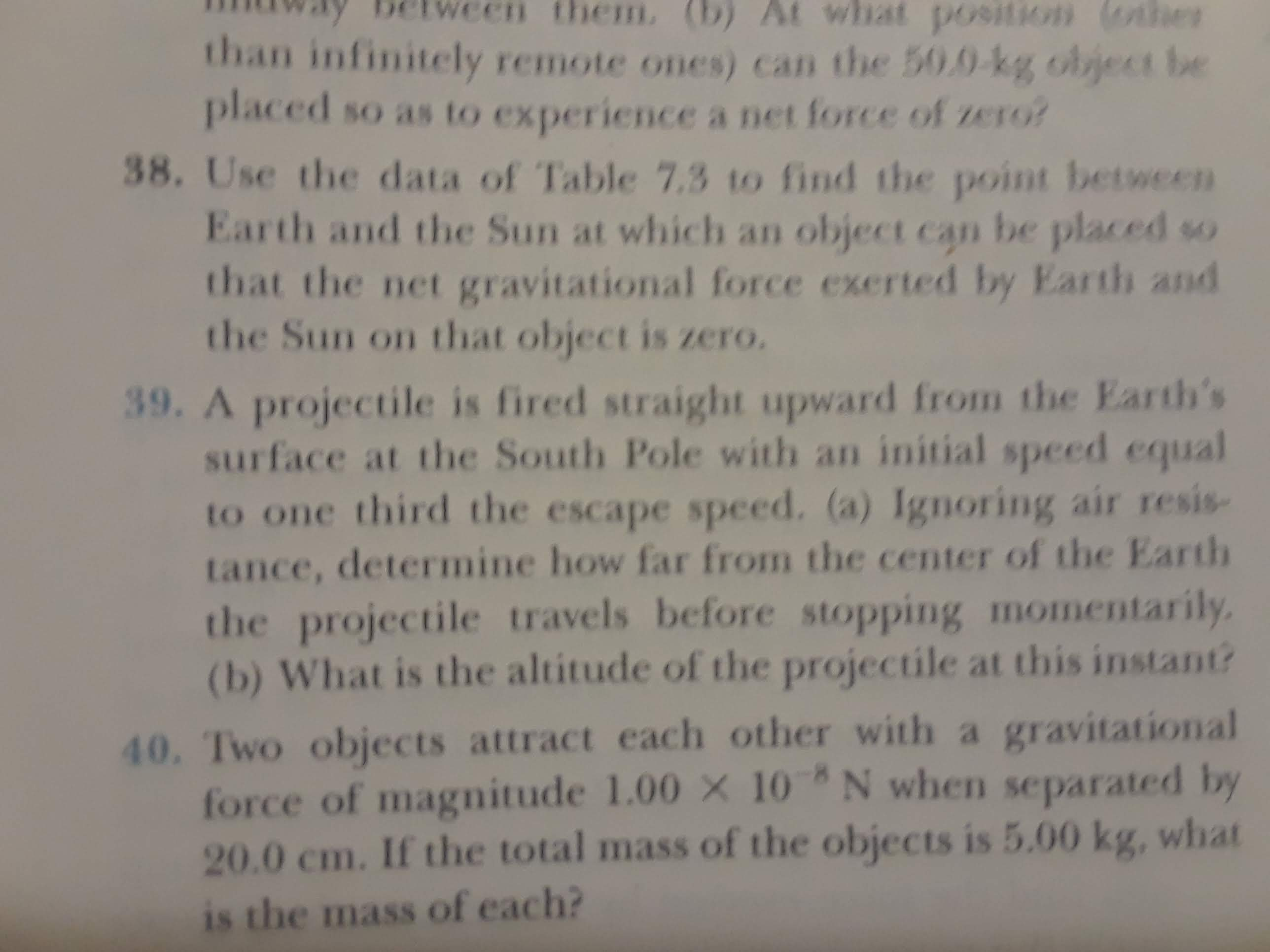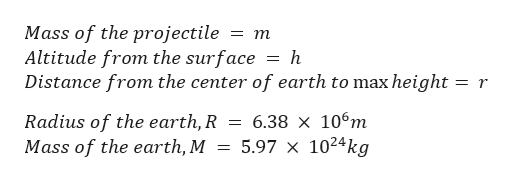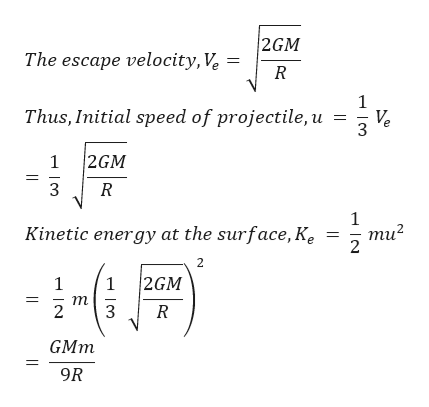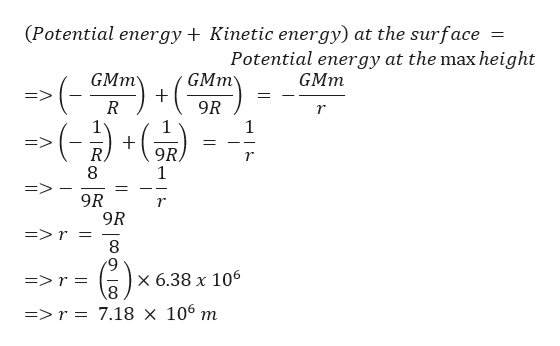# them. (b) At what po9ition (othhethan infinitely remote ones) can the 50.0-kg object beplaced so as to experience a net force of zero?38. Use the data of Table 7.3 to find the point betweenEarth and the Sun at which an object can be placed sothat the net gravitational force exerted by Earth andthe Sun on that object is zero.39. A projectile is fired straight upward from the Earth'ssurface at the South Pole with an initial speed equalto one third the escape speed. (a) Ignoring air resis-tance, determine how far from the center of the Earththe projectile travels before stopping momentarily(b) What is the altitude of the projectile at this instant?40. Two objects attract each other with a gravitationalforce of magnitude 1.00 X 10 N when separated by20.0 cm. If the total mass of the objects is 5.00 kg, whatis the mass of each?

Question
3 viewshelp_outlineImage Transcriptionclosethem. (b) At what po9ition (othhe than infinitely remote ones) can the 50.0-kg object be placed so as to experience a net force of zero? 38. Use the data of Table 7.3 to find the point between Earth and the Sun at which an object can be placed so that the net gravitational force exerted by Earth and the Sun on that object is zero. 39. A projectile is fired straight upward from the Earth's surface at the South Pole with an initial speed equal to one third the escape speed. (a) Ignoring air resis- tance, determine how far from the center of the Earth the projectile travels before stopping momentarily (b) What is the altitude of the projectile at this instant? 40. Two objects attract each other with a gravitational force of magnitude 1.00 X 10 N when separated by 20.0 cm. If the total mass of the objects is 5.00 kg, what is the mass of each? fullscreen
check_circle

Step 1

Given:help_outlineImage TranscriptioncloseMass of the projectile Altitude from the surface Distance from the center of earth to max height = r = m h = 6.38 x 106m 5.97 x 1024kg Radius of the earth, R Mass of the earth, M fullscreen
Step 2

We know:help_outlineImage Transcriptionclose2GM The escape velocity, Ve 1 Thus, Initial speed of projectile, u Ve 3 2GM 1 3 1 Kinetic energy at the surface, Ke ти? 2 2GM 1 т 2 3 GMm 9R fullscreen
Step 3

(a) The projectile...help_outlineImage Transcriptionclose(Potential energy Kinetic energy) at the surface Potential energy at the max height GMm (_GMm -() GMm 9R 1 R 9R 1 9R 9R =>r = 8 9 r х 6.38 х 106 8 =>r = 7.18 x 106 m fullscreen

### Want to see the full answer?

See Solution

#### Want to see this answer and more?

Solutions are written by subject experts who are available 24/7. Questions are typically answered within 1 hour.*

See Solution
*Response times may vary by subject and question.
Tagged in

### Centre of Mass and Centre of Gravity